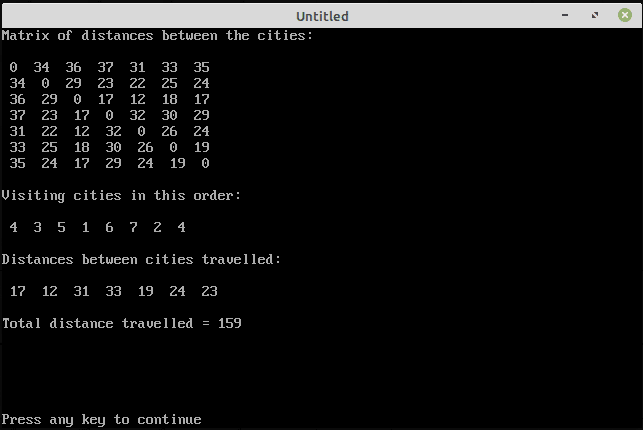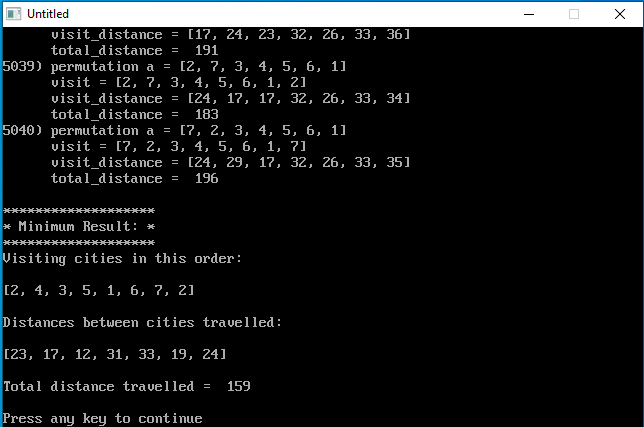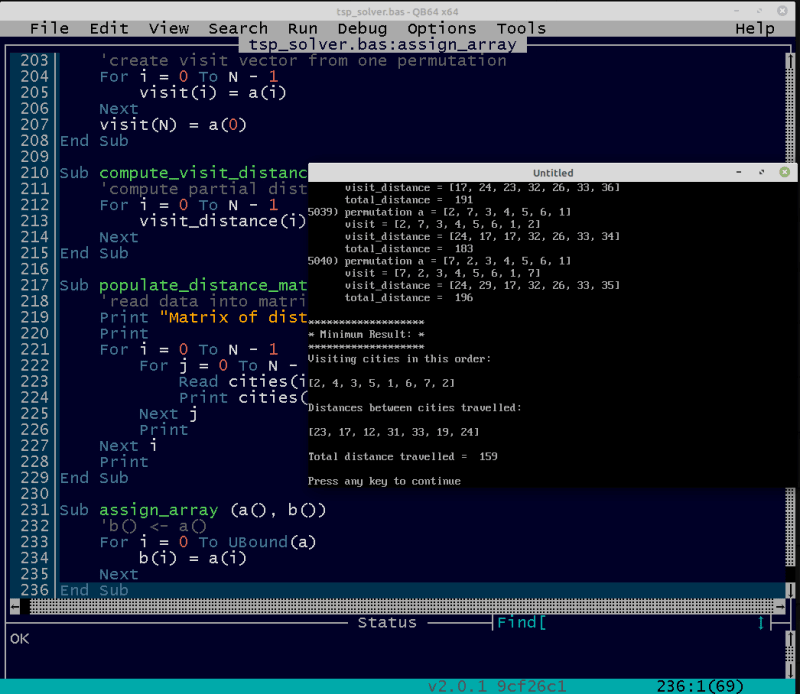×
INTELLIGENT WORK FORUMS
FOR COMPUTER PROFESSIONALS

Are you a
Computer / IT professional?
Join Tek-Tips Forums!
• Talk With Other Members
• Be Notified Of Responses
• Keyword Search
Favorite Forums
• Automated Signatures
• Best Of All, It's Free!

*Tek-Tips's functionality depends on members receiving e-mail. By joining you are opting in to receive e-mail.

#### Posting Guidelines

Promoting, selling, recruiting, coursework and thesis posting is forbidden.

# Travelling Salesman Problem in QBasic

## Travelling Salesman Problem in QBasic

### RE: Travelling Salesman Problem in QBasic

Hi pya,

Here is an example:

#### CODE

'Travelling Salesman Problem

'number of cities
N = 7

Dim cities(N, N)
Dim visit(N + 1)
Dim visit_distance(N)

Print "Matrix of distances between the cities:"
Print
For i = 1 To N
For j = 1 To N
Print cities(i, j);
Next j
Print
Next i
Print

Print "Visiting cities in this order:"
Print
For i = 1 To N + 1
Print visit(i);
Next i
Print
Print

'compute partial distances
Print "Distances between cities travelled:"
Print
For i = 1 To N
visit_distance(i) = cities(visit(i), visit(i + 1))
Print visit_distance(i);
Next i
Print
Print

'compute sum of distances
total_distance = 0
For i = 1 To N
total_distance = total_distance + visit_distance(i)
Next i
Print "Total distance travelled ="; total_distance

'---------------------------------------------------------------------
'Matrix contains distances between the cities
Distance_Matrix:
Data 0,34,36,37,31,33,35
Data 34,0,29,23,22,25,24
Data 36,29,0,17,12,18,17
Data 37,23,17,0,32,30,29
Data 31,22,12,32,0,26,24
Data 33,25,18,30,26,0,19
Data 35,24,17,29,24,19,0

'Vector contains the order in which to visit the cities
Visit_vector:
Data 4,3,5,1,6,7,2,4 

Output:### RE: Travelling Salesman Problem in QBasic

(OP)
Hi Mikrom,

I think I did not make myself clear.

I am trying to find the shortest route from any city (example 4) back to 4, while going through ALL cities exactly once.

Travelling Salesman Problem – Qbasic
5 'Travelling Salesman Problem

10 'number of cities
20 N = 10

30 Dim cities(N, N)
40 Dim visit(N + 1)
50 Dim visit_distance(N)

70 Print "Matrix of distances between the cities:"
80 Print
90 For i = 1 To N
100 For j = 1 To N
120 Print cities(i, j);
130 Next j
140 Print
150 Next i
160 Print

180 Print "Visiting cities in this order:"
190 Print
200 For i = 1 To N + 1
220 Print visit(i);
230 Next i
240 Print
250 Print

260 START = TIMER
270 'compute partial distances
280 Print "Distances between cities travelled:"
290 Print
300 For i = 1 To N
310 visit_distance(i) = cities(visit(i), visit(i + 1))
320 Print visit_distance(i);
330 Next i
340 Print
350 Print

360 'compute sum of distances
370 total_distance = 0
380 For i = 1 To N
390 total_distance = total_distance + visit_distance(i)
400 Next i
410 Print "Total distance travelled ="; total_distance
420 ELAPSED = TIMER – START
430 PRINT “elapsed time is” ; ELAPSED, “seconds”

440 '---------------------------------------------------------------------
450 'Matrix contains distances between the cities
460 Distance_Matrix:
470 Data 0,34,36,37,31,33,35,21,18,30
480 Data 34,0,29,23,22,25,24,31,20,27
490 Data 36,29,0,17,12,18,17,24,22,13
500 Data 37,23,17,0,32,30,29,16,33,29
510 Data 31,22,12,32,0,26,24,18,17,18
520 Data 33,25,18,30,26,0,19,20,18,22
530 Data 35,24,17,29,24,19,0,19,25,30
540 Data 21,31,24,16,18,20,19,0,21,24
550 Data 18,20,22,33,17,18,25,22,0,19
560 Data 30,27,13,29,18,22,30,24,19,0

700 'Vector contains the order in which to visit the cities
710 Visit_vector:
720 Data 4,3,5,1,6,7,2,4, 8,9,10

Your code, takes the visit vector, line 720, as input data.
I need to find the minimum route and then output the visit vector as the shortest route from city 4 back to 4, after visiting 1, 2, 3, 5, 6, 7,8,9,10,4 in any order which gives the shortest path.
Please see attached xls for 10 cities.

Seven cities will require enumerating 7! routes; 10 cities will require analysing 10! routes to find the shortest.

Thanx & Regards

Pervez

PS: I think I will take my star back :)

### RE: Travelling Salesman Problem in QBasic

Hi Pya,

In the excel sheet for 7 cities you posted the visit vector was hard coded. I didn't found any computation how you computed it. So I hard coded it too.
To compute it like you want, one first need to solve how to generate all 7! permutations. I don't know it yet. Maybe we will find an algorithm.

### RE: Travelling Salesman Problem in QBasic

Hi pya,

I found an algorithm how to compute all permutations: see QuickPerm algorithm here https://www.quickperm.org
Then I could use it for programming TSP.

tsp_solver.bas

#### CODE

'Travelling Salesman Problem

'number of cities
Const N = 7

Dim cities(N - 1, N - 1)
Dim visit(N)
Dim minimum_visit(N)
Dim visit_distance(N - 1)
Dim minimum_visit_distance(N - 1)
Dim a(N - 1)
Dim p(N)

Call populate_distance_matrix(cities())

output_file$= "tsp_solver.txt" Open output_file$ For Output As #1

For i = 0 To N - 1
a(i) = i + 1
Next

For i = 0 To N
p(i) = i
Next

output_line$= "Given number of cities:" Print output_line$
Print #1, output_line$output_line$ = "a = " + int_array_to_string$(a()) Print output_line$
Print #1, output_line$output_line$ = "All permutations:"
Print output_line$Print #1, output_line$

minimum_distance = 0
'-- QuickPerm algorithm
i = 0
nr = 1
Do While i < N
p(i) = p(i) - 1
j = 0
If i Mod 2 = 1 Then
j = p(i)
End If
Swap a(i), a(j)
i = 1
Do While p(i) = 0
p(i) = i
i = i + 1
Loop

'#-- processing one permutation
'create visit vector from permutation a()
Call permutation_to_visit(a(), visit())
'compute vector of distances between cities travelled
Call compute_visit_distances(cities(), visit(), visit_distance())

'compute total distance travelled
total_distance = total_distance_travelled(visit_distance())

'-- print visit info
Print Using "####)"; nr,
Print #1, Using "####)"; nr,

output_line$= " " _ + "permutation a = " + int_array_to_string$(a())
Print output_line$Print #1, output_line$

output_line$= " " _ + "visit = " + int_array_to_string$(visit())
Print output_line$Print #1, output_line$

output_line$= " " _ + "visit_distance = " _ + int_array_to_string$(visit_distance())
Print output_line$Print #1, output_line$

output_line$= " " _ + "total_distance = " + str$(total_distance)
Print output_line$Print #1, output_line$
'-- end of printing visit info

'check minimum
If nr = 1 Then
minimum_distance = total_distance
End If

If total_distance < minimum_distance Then
minimum_distance = total_distance
Call assign_array(visit(), minimum_visit())
Call assign_array(visit_distance(), minimum_visit_distance())
output_line$= " * ==> Less value found !" Print output_line$
Print #1, output_line$End If '#-- processing end nr = nr + 1 Loop Print Print #1, "" 'print result output_line$ = "*******************"
Print output_line$Print #1, output_line$

output_line$= "* Minimum Result: *" Print output_line$
Print #1, output_line$output_line$ = "*******************"
Print output_line$Print #1, output_line$

output_line$= "Visiting cities in this order:" Print output_line$
Print #1, output_line$Print Print #1, "" output_line$ = int_array_to_string$(minimum_visit()) Print output_line$
Print #1, output_line$Print Print #1, "" output_line$ = "Distances between cities travelled:"
Print output_line$Print #1, output_line$

Print
Print #1, ""

output_line$= int_array_to_string$(minimum_visit_distance())
Print output_line$Print #1, output_line$

Print
Print #1, ""

output_line$= "Total distance travelled = " + Str$(minimum_distance)
Print output_line$Print #1, output_line$

'close output file
Close #1

'---------------------------------------------------------------------
'Data
'---------------------------------------------------------------------
'Matrix contains distances between the cities
'Distance_Matrix:
'Data 0,34,36
'Data 35,20,29
'Data 40,29,10

Distance_Matrix:
Data 0,34,36,37,31,33,35
Data 34,0,29,23,22,25,24
Data 36,29,0,17,12,18,17
Data 37,23,17,0,32,30,29
Data 31,22,12,32,0,26,24
Data 33,25,18,30,26,0,19
Data 35,24,17,29,24,19,0

'---------------------------------------------------------------------
'Functions and Subroutines
'---------------------------------------------------------------------
Function int_array_to_string$(a()) res$ = "["
For i = 0 To UBound(a)
res$= res$ + LTrim$(Str$(a(i)))
If i < UBound(a) Then
res$= res$ + ", "
End If
Next i
res$= res$ + "]"
int_array_to_string$= res$
End Function

Function total_distance_travelled (visit_distance())
total_distance = 0
For i = 0 To N - 1
total_distance = total_distance + visit_distance(i)
Next
total_distance_travelled = total_distance
End Function

Sub permutation_to_visit (a(), visit())
'create visit vector from one permutation
For i = 0 To N - 1
visit(i) = a(i)
Next
visit(N) = a(0)
End Sub

Sub compute_visit_distances (cities(), visit(), visit_distance())
'compute partial distances
For i = 0 To N - 1
visit_distance(i) = cities(visit(i) - 1, visit(i + 1) - 1)
Next
End Sub

Sub populate_distance_matrix (cities())
Print "Matrix of distances between the cities:"
Print
For i = 0 To N - 1
For j = 0 To N - 1
Print cities(i, j);
Next j
Print
Next i
Print
End Sub

Sub assign_array (a(), b())
'b() <- a()
For i = 0 To UBound(a)
b(i) = a(i)
Next
End SubHowever you cannot see all 5040 permutation on the QB64 screen, so the program creates in the same directory the log file tsp_solver.txt which you can open with notepad and you can see all computation steps

tsp_solver.txt

#### CODE

Given number of cities:
a = [1, 2, 3, 4, 5, 6, 7]
All permutations:
1) permutation a = [1, 2, 3, 4, 5, 6, 7]
visit = [1, 2, 3, 4, 5, 6, 7, 1]
visit_distance = [34, 29, 17, 32, 26, 19, 35]
total_distance =  192
2) permutation a = [2, 1, 3, 4, 5, 6, 7]
visit = [2, 1, 3, 4, 5, 6, 7, 2]
visit_distance = [34, 36, 17, 32, 26, 19, 24]
total_distance =  188
* ==> Less value found !

...
...
...
...

5039) permutation a = [2, 7, 3, 4, 5, 6, 1]
visit = [2, 7, 3, 4, 5, 6, 1, 2]
visit_distance = [24, 17, 17, 32, 26, 33, 34]
total_distance =  183
5040) permutation a = [7, 2, 3, 4, 5, 6, 1]
visit = [7, 2, 3, 4, 5, 6, 1, 7]
visit_distance = [24, 29, 17, 32, 26, 33, 35]
total_distance =  196

*******************
* Minimum Result: *
*******************
Visiting cities in this order:

[2, 4, 3, 5, 1, 6, 7, 2]

Distances between cities travelled:

[23, 17, 12, 31, 33, 19, 24]

Total distance travelled =  159 

### RE: Travelling Salesman Problem in QBasic

(OP)
Hi Mikrom,

Will try it out later.

If it works I will give you another star.
If it does not I will take back the first star :)

Thanx

Pervez

### RE: Travelling Salesman Problem in QBasic

Hi pya,

I tested it on several cases, the largest case was with ten cities and it always calculated a result### RE: Travelling Salesman Problem in QBasic

(OP)
Hi mikrom,

When you have time can you please look at the ERROR I get when running your code.

Also, I do not understand Lines 2320 - 2360.

The Distance Matrix lines 2370 - 2384 I recognise as the distance between cities 1 to 7.

But what do lines 2320 - 2360 represent?

Regards

Pervez

PS: No urgency on this issue.

### RE: Travelling Salesman Problem in QBasic

Hi pya,

#### Quote (pya)

When you have time can you please look at the ERROR I get when running your code.
...
Attached is the compilelog ...

In the compile log you attached are only these lines

#### CODE

In file included from qbx.cpp:2120:0:
..\\temp\\main.txt:1285:1: error: 'LABEL_2700' does not name a type
compilation terminated due to -Wfatal-errors. 
From this I can not determine what the cause of the error is.
Maybe you changed something in the program improperly.
The code I posted, I tested before in QB64 on two PCs: my home PC with Linux and my work PC with Windows 10. It runs successfully on both.
First try to run the program as I posted it, without changing anything.
I also noticed from the screenshot you posted that you have a different version of QB64 than me. You have a 32 bit version (QB64 x32) and I have a 64 bit version (QB64 x64).

#### Quote (pya)

Also, I do not understand Lines 2320 - 2360.
I don't know what line numbers you mean.
The program I posted has only 236 lines - see the screenshot:### RE: Travelling Salesman Problem in QBasic

(OP)
Hi mikrom,

Mea Culpa!

I must have screwed up during line numbering.

Pervez

### RE: Travelling Salesman Problem in QBasic

(OP)
Hi mikrom,

I ran your code for 10 cities and it worked fine.

But, I have a couple of questions and would be grateful if you could answer at your convenience.

1. I do not understand the first set of Distance Matrix - DATA 0,34,36 and the following 2 lines.

2. My 2nd question is where is the right place to put a TIMER?
I start the timer after the initial 7 DIM statements and end it just before Functions and Subroutines

My aim is to calculate the computing time only and not include any printing or output time.

Pervez

'Data
'---------------------------------------------------------------------
'Matrix contains distances between the cities
'Distance_Matrix:
'Data 0,34,36
'Data 35,20,29
'Data 40,29,10

Distance_Matrix:
Data 0,34,36,37,31,33,35,21,18,30
Data 34,0,29,23,22,25,24,31,20,27
Data 36,29,0,17,12,18,17,24,22,13
Data 37,23,17,0,32,30,29,16,33,29
Data 31,22,12,32,0,26,24,18,17,18
Data 33,25,18,30,26,0,19,20,18,22
Data 35,24,17,29,24,19,0,19,25,30
Data 21,31,24,16,18,20,19,0,21,24
Data 18,20,22,33,17,18,25,22,0,19
Data 30,27,13,29,18,22,30,24,19,0

ELAPSED = TIMER – START
PRINT “elapsed time is”; ELAPSED, “secs”

'---------------------------------------------------------------------
'Functions and Subroutines
'---------------------------------------------------------------------

### RE: Travelling Salesman Problem in QBasic

Hi pya,

#### Quote:

I do not understand the first set of Distance Matrix - DATA 0,34,36 and the following 2 lines.
As you could see these lines begin with ' and are set on comments. It was only a small example of three cities on which I tested the program during development. You can delete these lines.

#### Quote:

My 2nd question is where is the right place to put a TIMER?
I start the timer after the initial 7 DIM statements and end it just before Functions and Subroutines
I would START the timer as you have done and END it after the main program is over i.e. after these lines

#### CODE

'close output file
Close #1 

#### Quote:

My aim is to calculate the computing time only and not include any printing or output time.
Then make your own modification of the program, where you remove:
commands for opening and closing output file
all commands needed for printing the intermediate results on the screen and in the file, i.e. statements which begin with output_line$and Print ### RE: Travelling Salesman Problem in QBasic (OP) Hi mikrom, One last question: How do I insert spaces between the brackets in the output? ( 2, .....7, 2 ) and ( 23, .... 19, 24 ) At my age I can get confused if the output is like (2, ....7, 2) and (23, .....19,24) I modified a few lines but got no change. Regards Pervez ### RE: Travelling Salesman Problem in QBasic You can insert the spaces in the function int_array_to_string$

#### Red Flag This Post

Please let us know here why this post is inappropriate. Reasons such as off-topic, duplicates, flames, illegal, vulgar, or students posting their homework.

#### Red Flag Submitted

Thank you for helping keep Tek-Tips Forums free from inappropriate posts.
The Tek-Tips staff will check this out and take appropriate action.

Close Box

# Join Tek-Tips® Today!

Join your peers on the Internet's largest technical computer professional community.
It's easy to join and it's free.

Here's Why Members Love Tek-Tips Forums:

•Talk To Other Members
• Notification Of Responses To Questions
• Favorite Forums One Click Access
• Keyword Search Of All Posts, And More...

Register now while it's still free!